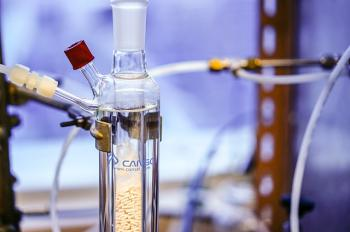# Chemical thermodynamicsChemical thermodynamics is the study of the interrelation of heat and work with chemical reactions or with physical changes of state within the limits of the laws of thermodynamics. Chemical thermodynamics involves not only laboratory measurements of various thermodynamic properties, but also the application of mathematical methods to the study of chemical issues and the spontaneity of processes.

The structure of chemical thermodynamics is based on the first two laws of thermodynamics. From the first and second laws of thermodynamics, four equations called "fundamental Gibbs equations" can be derived. From these four, a multitude of equations can be derived that relate the thermodynamic properties of the thermodynamic system using relatively simple mathematics. This describes the mathematical framework of chemical thermodynamics.

Chemical thermodynamics is in close contact with chemistry sections such as

• analytic chemistry
• electrochemistry
• colloidal chemistry

## Objective of chemical thermodynamics

The main objective of chemical thermodynamics is the establishment of a criterion to determine the viability or spontaneity of a given transformation. In this way, chemical thermodynamics is normally used to predict the energy exchanges that occur in the following processes:

• Chemical reactions
• Phase changes
• The formation of solutions.

The following state functions are of primary concern in chemical thermodynamics:

• Internal energy (U)
• Enthalpy (h). Enthalpy is a physical quantity defined in the field of classical thermodynamics to measure the maximum energy of a thermodynamic system theoretically capable of being removed from it in the form of heat. It is particularly useful to understand and describe isobaric processes: constant pressure, the enthalpy variation is directly associated with the energy received by the system in the form of heat, these are easily quantifiable with calorimeters.
• Entropy (S). Entropy is a thermodynamic quantity that explains the energy that can not be used in a thermodynamic process. It is a function of a state of extensive character and its value grows in an isolated system, according to the second law of thermodynamics. It can be interpreted as the state of disorder of a system. The positive variation of entropy indicates the natural sense in which any event occurs in an isolated system.
• Gibbs free energy (G). In thermodynamics, the free energy of Gibbs (or free enthalpy) is a mathematical expression, more specifically a function of extended state, which provides the condition of balance and spontaneity for any process that takes place under constant pressure (for example, many reactions) . chemical products). The changes in free energy are symbolized as ΔG and represent the energy available for useful chemical work.

Most identities in chemical thermodynamics arise from the application of the first law of thermodynamics and the second law of thermodynamics, in particular the law of conservation of energy, to these state functions.

## Laws of thermodynamics

In short, the three laws of thermodynamics announce the following:

The energy of the universe is constant.
In any spontaneous process, there is always an increase in the entropy of the universe.
The entropy of a perfect crystal (well ordered) at 0 Kelvin is zero.

The laws of thermodynamics describe, in principle, the private transport of heat and work in thermodynamic processes. However, from its conception, these laws of physics became the most important of all physics and other sciences related to thermodynamics. They are often associated with concepts that are too far removed from what their statements contain.

Laws of thermodynamics

0. Zero law of thermodynamics

one. First Law of Thermodynamics

two. Second law of thermodynamics

3. Third law of thermodynamics

The first principle that was established was the second law of thermodynamics, as formulated by Sadi Carnot in 1824. The 1860 already established two "principles" of thermodynamics with the works of Rudolf Clausius and William Thomson, Lord Kelvin. Over time, these principles have become "laws." In 1873, for example, Willard Gibbs claimed that there were two absolute laws of thermodynamics in his graphical methods in fluid thermodynamics. Currently a total of four laws are enunciated. In the last 80 years, some authors have suggested other laws, but none of them was accepted unanimously.

## History of chemical thermodynamics

The development of chemical thermodynamics developed simultaneously in two ways: thermochemistry and thermodynamics.

The emergence of thermochemistry as an independent science should be considered as the discovery by Herman Ivanovich Hess, professor at the University of Petersburg, the relationship between the thermal effects of chemical reactions, the laws of Hess.

In 1867, on the basis of thermochemistry, Marselen Berthelot proposed one of the first theories of chemical affinity, explaining the direction of the flow of chemical reactions.

The general achievements of thermodynamics, as part of theoretical chemistry, reflected in the study of entropy, led to the emergence of another method of research, unrelated to the theory of chemical affinity tested negatively.

The foundations of classical chemical thermodynamics were established in the work of Josiah Willard Gibbs "On the balance of heterogeneous substances" (1878), in which a graphical method was developed to represent the states of a substance, the method of potentials thermodynamics and the rule of phases.

Gibbs' methods, also developed by Pierre Dueme, gave a great boost to the development of thermodynamic applications, since they were much simpler than the method of circular processes, which required inventing hypothetical processes that closed certain processes in a circular one.

valoración: 3 - votos 1

Last review: October 30, 2018

Back